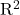## A Sociology student investigated the association between a country’s Literacy Rate and Life Expectancy, and then drew the conclusions listed

Question

A Sociology student investigated the association between a country’s Literacy Rate and Life Expectancy, and then drew the conclusions listed below. Explain why each statement is incorrect. (Assume that all the calculations were done properly.)
a) The $$R^2$$ of 64% means that the Literacy Rate determines 64% of the Life Expectancy for a country.
b) The slope of the line shows that an increase of 5% in Literacy Rate will produce a 2-year improvement in Life Expectancy.

in progress 0
6 months 2021-08-02T07:59:09+00:00 1 Answers 29 views 0

a).does not determine the rate at which the variable explains another, but it explains the amount the amount of variability in one of the variables as explained by the other variable.